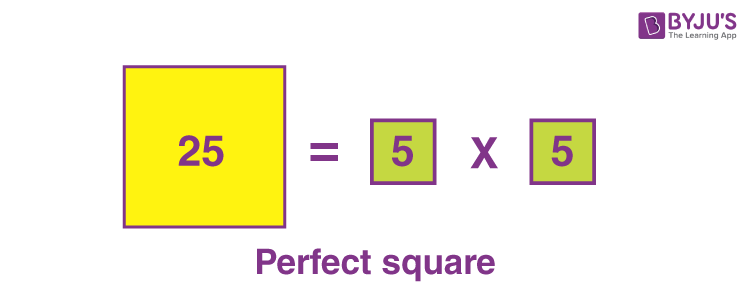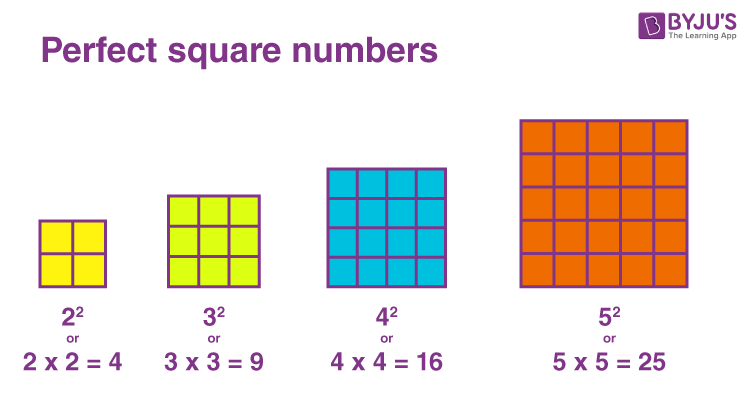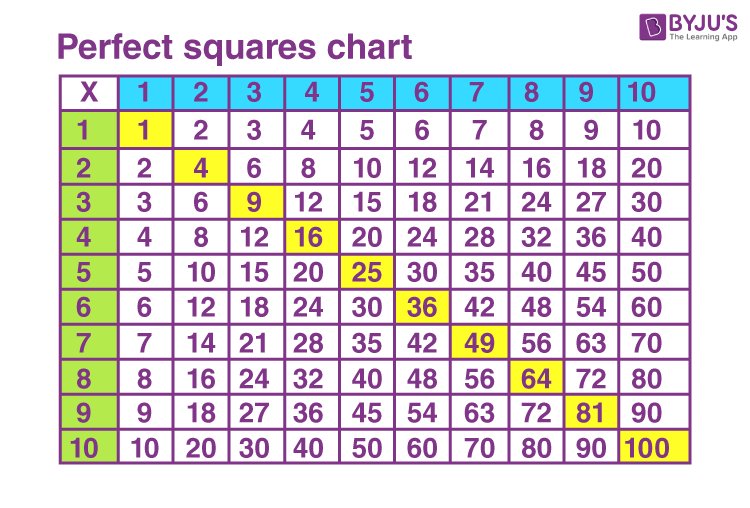# Perfect Squares

In mathematics, we might have come across different types of numbers such as even, odd, prime, composite, etc. However, there is a particular type of number, i.e. a perfect square. These can be identified and expressed with the help of factorisation of a number. In this article, you will learn the definition of perfect square numbers, notation, the list of these numbers between 1 and 100 and so on.

## Perfect Squares Definition

An integer that can be expressed as the square of another integer is called a perfect square. In other words, it is defined as the product of some integer with itself.## Perfect Square Numbers

We know that the square of a number is that number times itself. In other words, the perfect squares are the squares of the whole numbers such as 1 or 12, 4 or 22, 9 or 32, 16 or 42, 25 or 52 and so on.Also, get the perfect square calculator here.

## Perfect Squares from 1 to 100

Below shows the list of perfect squares from 1 to 100 along with their factors (product of integers).

 Perfect square numbers from 1 to 100 1 = 1 × 1 = 12 4 = 2 × 2 = 22 9 = 3 × 3 = 32 16 = 4 × 4 = 42 25 = 5 × 5 = 52 36 = 6 × 6 = 62 49 = 7 × 7 = 72 64 = 8 × 8 = 82 81 = 9 × 9 = 92 100 = 10 × 10 = 102

### Perfect Squares List

The perfect squares table is given below in terms of squares of numbers from 1 to 50.

 1 = 12 441 = 212 1681 = 412 4 = 22 484 = 222 1764 = 422 9 = 32 529 = 232 1849 = 432 16 = 42 576 = 242 1936 = 442 25 = 52 625 = 252 2025 = 452 36 = 62 676 = 262 2116 = 462 49 = 72 729 = 272 2209 = 472 64 = 82 784 = 282 2304 = 482 81 = 92 841 = 292 2401 = 492 100 = 102 900 = 302 2500 = 502 121 = 112 961 = 312 2601 = 512 144 = 122 1024 = 322 2704 = 522 169 = 132 1089 = 332 2809 = 532 196 = 142 1156 = 342 2916 = 542 225 = 152 1225 = 352 3025 = 552 256 = 162 1296 = 362 3136 = 562 289 = 172 1369 = 372 3249 = 572 324 = 182 1444 = 382 3364 = 582 361 = 192 1521 = 392 3481 = 592 400 = 202 1600 = 402 3600 = 602

From this we can derive the formula to get the difference between any perfect square number and its predecessor. This is given by the equation,

n2 − (n − 1)2 = 2n − 1

However, it is possible to count the number of square numbers using the formula,

n2 = (n − 1)2 + (n − 1) + n

### Perfect Squares Examples

Perfect square numbers are not only limited to the numerals but also exist in algebraic identities and polynomials. These can be identified with the help of a factorisation technique.

Algebraic identities as perfect squares:

a2 + 2ab + b2 = (a + b)2

a2 – 2ab + b2 = (a – b)2

Polynomials as perfect squares:

Let us take the polynomial x2 + 10x + 25.

Now, factorise the polynomial.

x2 + 10x + 25 = x2 + 5x + 5x + 25

= x(x + 5) + 5(x + 5)

= (x + 5)(x + 5)

= (x + 5)2

Let us take another example:

x2 – 12x + 36 = x2 – 6x – 6x + 36

= x(x – 6) – 6(x – 6)

= (x – 6)(x – 6)

= (x – 6)2

From the above examples, we can say that x2 + 10x + 25 and x2 – 12x + 36 are called perfect square trinomials.

### Perfect Squares Chart### How many Perfect Squares are between 1 and 100?

There are eight perfect squares between 1 and 100 (i.e., excluding 1 and 100).

They are 4, 9, 16, 25, 36, 49, 64 and 81.

However, there are ten perfect squares from 1 to 10. They are 1, 4, 9, 16, 25, 36, 49, 64, 81 and 100.

### How many Perfect Squares are between 1 and 1000?

There are 30 perfect squares between 1 and 1000. They are 4, 9, 16, 25, 36, 49, 64, 81, 100, 121, 144, 169, 196, 225, 256, 289, 324, 361, 400, 441, 484, 529, 576, 625, 676, 729, 784, 841, 900 and 961.

### Is 216 a perfect square?

A number is a perfect square or a square number if its square root is an integer, which means it is an integer’s product with itself. As we know, the square root of 216 is approximately equal to 14.697. Here, the square root of 216 is not an integer. Hence, it is clear that 216 is not a perfect square number.